# PSAT Math : How to factor a variable

## Example Questions

### Example Question #1 : Factoring

Factor the following variable

(x2 + 18x + 72)

(x + 18) (x + 72)

(x – 6) (x + 12)

(x + 6) (x + 12)

(x – 6) (x – 12)

(x + 6) (x – 12)

(x + 6) (x + 12)

Explanation:

You need to find two numbers that multiply to give 72 and add up to give 18

easiest way: write the multiples of 72:

1, 72

2, 36

3, 24

4, 18

6, 12: these add up to 18

(x + 6)(x + 12)

### Example Question #2 : Factoring

Factor 9x2 + 12x + 4.

(9x + 4)(9x + 4)

(3x + 2)(3x + 2)

(3x – 2)(3x – 2)

(3x + 2)(3x – 2)

(9x + 4)(9x – 4)

(3x + 2)(3x + 2)

Explanation:

Nothing common cancels at the beginning. To factor this, we need to find two numbers that multiply to 9 * 4 = 36 and sum to 12. 6 and 6 work.

So 9x2 + 12x + 4 = 9x2 + 6x + 6x + 4

Let's look at the first two terms and last two terms separately to begin with. 9x2 + 6x can be simplified to 3x(3x + 2) and 6x + 4 can be simplified into 2(3x + 2). Putting these together gets us

9x2 + 12x + 4

= 9x2 + 6x + 6x + 4

= 3x(3x + 2) + 2(3x + 2)

= (3x + 2)(3x + 2)

This is as far as we can factor.

### Example Question #3 : Factoring

If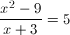, and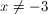, what is the value of?

–8

–6

8

0

6

8

Explanation:

The numerator on the left can be factored so the expression becomes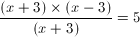, which can be simplified to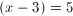Then you can solve forby adding 3 to both sides of the equation, so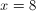### Example Question #4 : Factoring

Solve for x: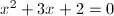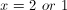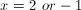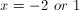Explanation:

First, factor.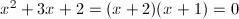Set each factor equal to 0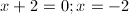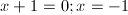Therefore,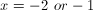### Example Question #5 : Factoring

When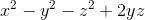is factored, it can be written in the form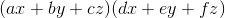, where,,,,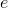, andare all integer constants, and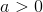.

What is the value of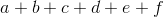?Explanation:

Let's try to factor x2 – y2 – z2 + 2yz.

Notice that the last three terms are very close to y2 + z2 – 2yz, which, if we rearranged them, would become y2 – 2yz+ z2. We could factor y2 – 2yz+ z2 as (y – z)2, using the general rule that p2 – 2pq + q2 = (p – q)2 .

So we want to rearrange the last three terms. Let's group them together first.

x2 + (–y2 – z2 + 2yz)

If we were to factor out a –1 from the last three terms, we would have the following:

x2 – (y2 + z2 – 2yz)

Now we can replace y2 + z2 – 2yz with (y – z)2.

x2 – (y – z)2

This expression is actually a differences of squares. In general, we can factor p2 – q2 as (p – q)(p + q). In this case, we can substitute x for p and (y – z) for q.

x2 – (y – z)= (x – (y – z))(x  + (y – z))

Now, let's distribute the negative one in the trinomial x – (y – z)

(x – (y – z))(x  + (y – z))

(x – y + z)(x + y – z)

The problem said that factoring x2 – y2 – z2 + 2yz would result in two polynomials in the form (ax + by + cz)(dx + ey + fz), where a, b, c, d, e, and f were all integers, and a > 0.

(x – y + z)(x + y – z) fits this form. This means that a = 1, b = –1, c = 1, d = 1, e = 1, and f = –1. The sum of all of these is 2.

### Example Question #6 : Factoring

Factor and simplify: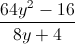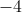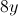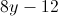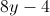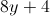Explanation: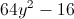is a difference of squares.

The difference of squares formula is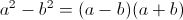.

Therefore,=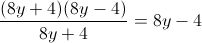.

### Example Question #7 : Factoring

Factor: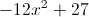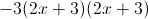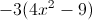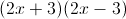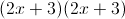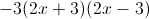Explanation:

We can first factor out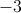:This factors further because there is a difference of squares: